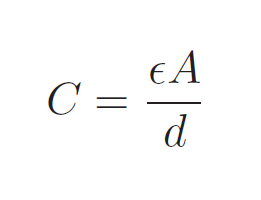# Capacitive level measurement

## Principle:

Capacitive level instrument uses the principle of capacitance equationC - capacitance E - Permittivity of dielectric material between plates. A - overlapping area of plates d - Distance between plates

## Construction:

There is a probe inserted at the middle of the tank which is a dielectric material. The probe form one electrode of the capacitor and the metal sheet at the walls form the other. Capacitance is measured across the probe and the metal plate on the wall.

The liquid inside the tank forms the dielectric material of the capacitor if the liquid is non-conductive. If the liquid is conductive then we have to cover the probe with a dielectric sheath to deliver a capacitance function.

## Working:

Analysing the capacitance equation, here in the setup of level measurement d-distance and A-area, both are constant. As area and distance are tank dimensions. When liquid raises the dielectric constant increases, thus capacitance also increases. Air has less dielectric value than liquid.

As the liquid level increases the capacitance increases and capacitance provides the direct relation with the level of the tank. The microprocessor attached to the sensors can calculate the level of the changing capacitance value.

## Application:

• Liquid level measurement

• As well powder and solid level measurement.

• Level measurement of liquid metals at a very high temperature and liquefied gases at low temperature.

• Inexpensive

• Low maintenance

• Contains no moving part

• Good range of measurement.

• Can be used for High temperature and High pressure applications.